# Cube and Dice Tricks for SSC and Railways Exams

In many exams like SSC CGL, SSC CHSL, RRB NTPC, etc., Cubes and Dice is one of the most important chapters. This type of question is asked under the visual reasoning section. This section measures the ability to understand and analyze information presented visually and then solve problems logically. We will get you acquainted with the various Cube and Dice Tricks. This article will help you to solve such questions in a quick manner and with good accuracy.

Attempt a free RRB NTPC Mock Here

## 1. Scope of the Topic for SSC and RRB

This topic is a regular topic in exams SSC CGL, SSC CHSL, RRB NTPC, etc. For SSC CGL, in tier I and tier II; for SSC CHSL in tier I and for RRB NTPC in 1st Stage Computer Based Test (CBT) and 2nd Stage Computer Based Test (CBT) the questions on cubed and dice are often asked.

The types of questions asked are:

i) Constructed and deconstructed dice

ii) Observations of an open die

iii) Finding the opposite side

iv) Odd one out

Generally, around 2 – 3 questions are asked. The difficulty level of these questions is easy or easy-moderate.

Check the detailed Syllabus here.

## 2. Dice – Basic Concepts

Die is a small, throwable object with marked sides that can rest in multiple positions. Dice is a cube. It has a different number placed on each side.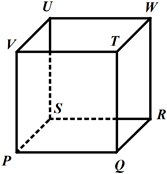### 2.1 Basic properties

1. There are six faces, eight vertices, and twelve edges in a cube.
2. Four faces are adjacent to one face.
3. There are three pairs of opposite faces.
4. Only three sides of a cube are visible at a time and these sides can never be on the opposite sides of each other.

### 2.2 Open Dice

The following diagram is of an open dice when it is flattened out.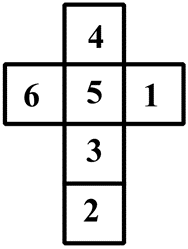• 2 is the top face of the cube.
• 5 is the bottom face of the cube.
• 4, 6, 1, and 3 are the side faces of the cube.
• Faces 6 and 1 are always opposite to each other.
• Faces 4 and 2; 5 and 2 do not have a common side since these faces are opposite to each other.

### 2.3 Observation of an Open Dice

Variations of an open die are as follows :

A)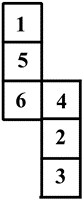Faces 1 and 6, faces 4 and 3, faces 5 and 2 are opposite to each other.

B)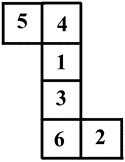Faces 1 and 6, faces 4 and 3, faces 5 and 2 are opposite to each other.

C)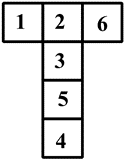Faces 1 and 6, faces 4 and 3, faces 5 and 2 are opposite to each other.

Register here and Access Various FREE Mocks.

## 3. Cube and Dice Tricks

To master various Cube and Dice Tricks, one should learn the two basic rules of cubes and dice. Most of the questions asked can be addressed by using these rules.

#### 3.1 Rule 1

If two dice are given in different orientations but one face is common. Then the remaining surfaces are opposite to each other.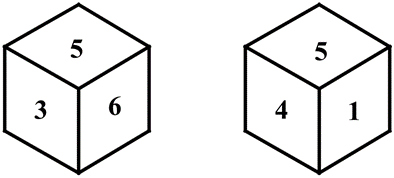Here, face 5 is the common face.

Faces 3, 6, 4, and 1 are adjacent to the face 2. Hence, face 2 is opposite to face 5 here.

Faces 3 and 4 and faces 6 and 1 are opposite to each other.

#### 3.2 Rule 2

If two dice are given in different orientations but two faces are common. If out of those two common faces one face is in the same position, then the faces which are not common are opposite to each other.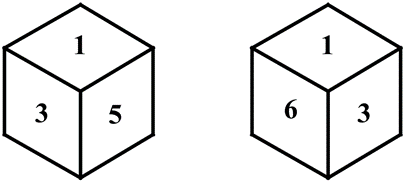Here, faces 1 and 3 are common faces.

Face 1 is at the same position.

Face 5 and 6 are not common.

Hence, faces 5 and 6 are opposite to each other.

## 4. Sample Questions – Cube and Dice Tricks

#### 4.1 Question 1

When the following figure is folded to form a cube, then which of the following shows a pair of faces opposite to each other?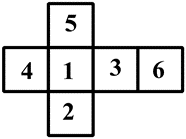(1) 2 – 3

(2) 4 – 6

(3) 5 – 6

(4) 5 – 2

(5) 4 – 2

Solution: Here, faces 1 and 6, faces 4 and 3, faces 5 and 2 are opposite to each other.

#### 4.2 Question 2

When the following figure is folded to form a cube, which of the following options can be the folded cube?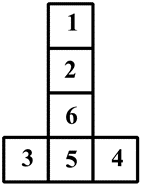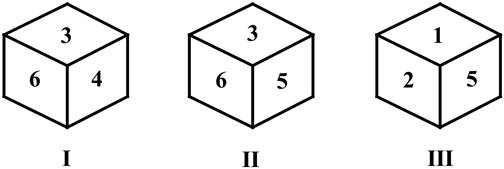(1) Only I

(2) Only II

(3) Only III

(4) Both I and II

(5) Both II and III

Solution: In the given flattened-out figure, faces 1 and 6, faces 4 and 3, faces 5 and 2 are opposite to each other.

In figure I faces 3 and 4 are adjacent to each other and in figure III, faces 2 and 5 are adjacent to each other.

Hence, figures I and III cannot be the folded cube.

In figure II, faces 6, 3, and 5 can be adjacent to each other. Figure II can be the folded cube.

Download free E-books here – Practice Questions and Concepts for Various Topics.

Being one of the important topics of the reasoning section in SSC and RRB examinations, much importance should be given to this topic. Candidates can perfect this topic by practicing more questions of different difficulty levels. Oliveboard provides an ample amount of mock tests and topic quizzes to perfect the topic. Candidates should also be aware of the fact that the types can be combined with each other to form mixed or miscellaneous questions which increase the difficulty level of the topic. Practicing such questions will be of great help to prepare for various examinations. All the best.